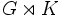# Complement to normal subgroup is isomorphic to quotient group

## Statement

Suppose$G$ is a group,$N$ is a normal subgroup and$H$ is a subgroup such that$N$ and$H$ are permutable complements:$NH = G$ and$N \cap H$ is trivial. Then,$G/N \cong H$.

In particular, any two permutable complements to$N$ are isomorphic to each other.

## Related facts

### Caveats

Note the following:

• If$N$ is a normal subgroup of$G$, it is not necessary that$N$ has a permutable complement in$G$. For instance, if$G$ is the cyclic group of order four and$N$ is a subgroup of order two,$N$ does not have a permutable complement. A normal subgroup that does have a permutable complement is termed a complemented normal subgroup. A subgroup that occurs as the permutable complement to a normal subgroup is termed a retract.
• Any lattice complement to$N$ in$G$ is also a permutable complement to$N$ in$G$. This is because any normal subgroup is permutable: its product with any subgroup is a subgroup. Further information: Equivalence of definitions of complemented normal subgroup

### Other related facts

• Complements to abelian normal subgroup are automorphic: If$N$ is an abelian normal subgroup of$G$ and$H, K$ are two permutable complements to$N$ in$G$, then there is an automorphism of$G$ that is the identity map on$N$ and sends$H$ to$K$. In particular,$H$ and$K$ are automorphic subgroups.
• Complements to normal subgroup need not be automorphic: If$N$ is a normal subgroup of and$H,K$ are two permutable complements to$N$ in$G$, there need not be an automorphism of$G$ sending$H$ to$K$.
• Retract not implies normal complements are isomorphic: This states that if$N$ and$M$ are two permutable complements to a subgroup$H$, and both$N$ and$M$ are normal, this does not imply that$N$ is isomorphic to$M$. In other words, interchanging the role of which subgroup is the normal one renders the result false.
• Schur-Zassenhaus theorem (normal Hall implies permutably complemented and Hall retract implies order-conjugate): This states that every normal Hall subgroup, and hence every normal Sylow subgroup, has a complement, and that any two such complements are conjugate subgroups in the whole group.
• Every group of given order is a permutable complement for symmetric groups: Any group of order$n$ occurs as a permutable complement to$\operatorname{Sym}(n-1)$ in$\operatorname{Sym}(n)$, via the embedding obtained by Cayley's theorem. This is a far cry from the fact that any two permutable complements to a normal subgroup are isomorphic.
• Semidirect product is not left-cancellative for finite groups: We can have finite groups$G,H,K$ such that$G \rtimes H \cong G \rtimes K$, but$H$ is not isomorphic to$K$. Note that the isomorphism between$G \rtimes H$ and$G \rtimes K$ must not map the normal subgroup$G$ to the normal subgroup$G$, otherwise we would contradict the result of this page.